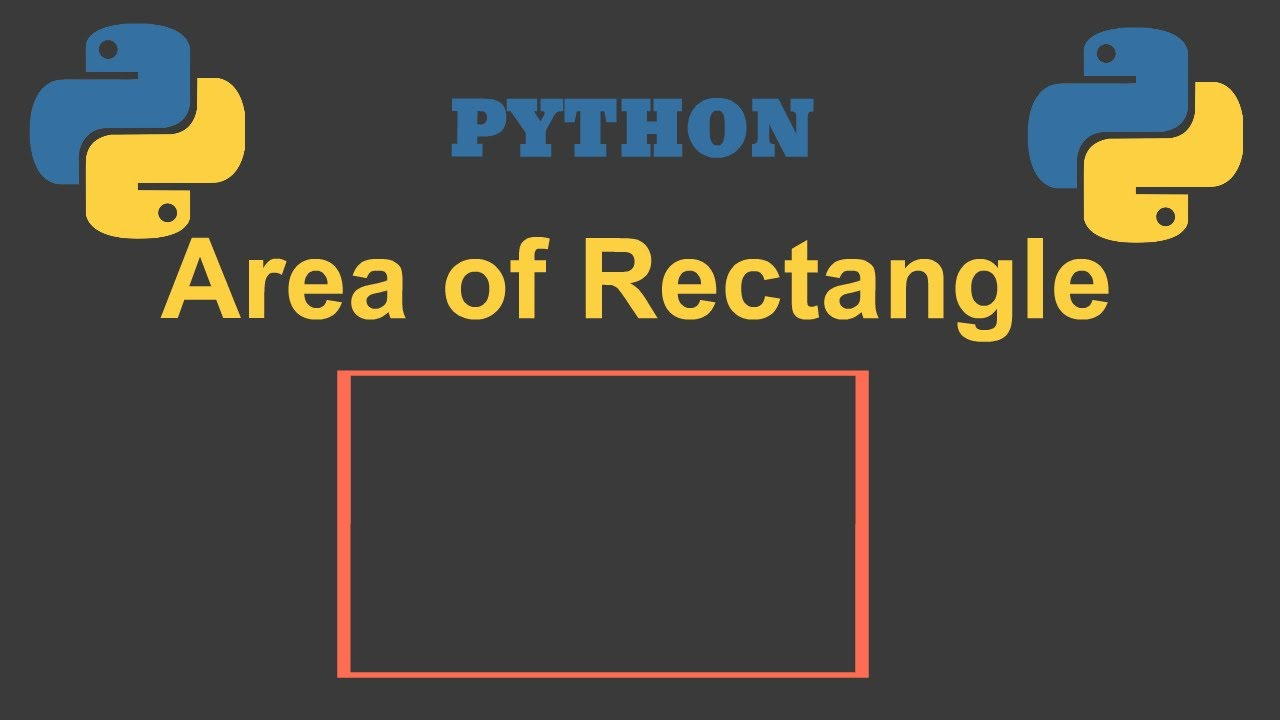# Python program to calculate area of rectangle||Python||Parvat Computer TechnologyProgram to obtain length and breadth of the rectangle and calculate its area.To input the length and breadth of a rectangle to calculate its area.Guys in thi...

Program to obtain length and breadth of the rectangle and calculate its area. To input the length and breadth of a rectangle to calculate its area. Guys in this video I try to show that how can we create a program to calculate the area of a rectangle. It is a special calculator created by python to measure the area of a rectangle. we can also find the area of the square by entering the same length and breadth of a square. 💵💵💵💵💵💵💵💵💵💵💵💵💵💵💵💵💵💵💵💵 AREA OF RECTANGLE =length * breadth 💵💵💵💵💵💵💵💵💵💵💵💵💵💵💵💵💵💵💵💵

GUYS IF THIS VIDEO IS HELPFUL TO YOU THAN LIKE, SHARE, AND SUBSCRIBE TO MY YOUTUBE CHANNEL. https://youtu.be/A5UDRG2Ca1M

## top 30 Python Tips and Tricks for Beginners

In this post, we'll learn top 30 Python Tips and Tricks for Beginners

## Guide to Python Programming Language

Guide to Python Programming Language